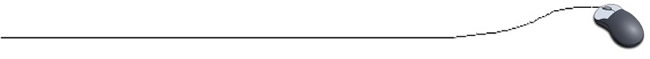Finding the Maximum or Minimum
(finding the highest (or lowest) value in an array)
 There are numerous algorithms that allow you to manipulate the information stored in an array.  Consider this method which allows you to determine the maximum value in an array of integers (could be easily adapted to find minimum value instead): //Method to find highest (maximum) value in array public static int maximumValue(int [ ] array) {      int length = array.length;  // establish size of array      int max = array;     // start with max = first element      for(int i = 1; i < length; i++)      {           if(array[i] > max)                 max = array[i];      }      return max;           // return highest value in array }

 Definition: An algorithm is a programming method that performs a specific job.